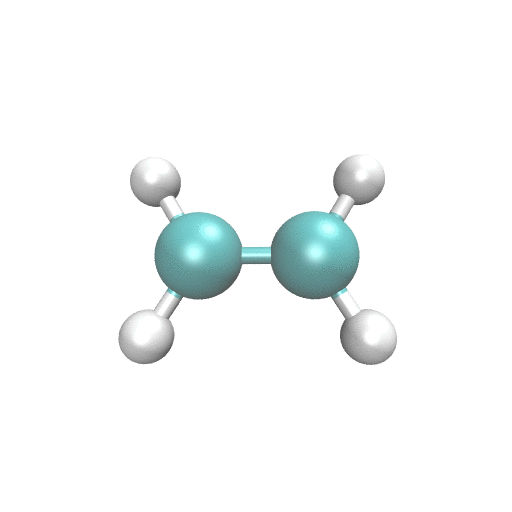Tutorial 3. Basics of running TeraChem

Learning objectives

• Run a simple GPU-based quantum chemistry calculation
• Get familiar with running calculations on computer clusters

Introduction

In the previous tutorial we have tried running a quantum chemistry calculation with a CPU-based program installed on your own computer. Here we will use TeraChem, the GPU-based quantum chemistry package. The software is installed on certain super computers with suitable GPU hardware, so we need to remote log in the super computers and run calculations there, as shown in the figure cited below:Figure 1.Schematic of typical architecture of a computer cluster Available from: https://en.wikipedia.org/wiki/Computer_cluster [accessed 9 Sep, 2020]

Unlike your own computer, there are many users on the supercomputer. If all users directly run the calculation at the same time, the computer may freeze or even crash. Besides, supercomputers are clusters of a large number of computers connected in the network, and each computer is known as a node. The node where you log in may not have the hardware you need, and directly running the calculation in your home directory may result in failure. Instead, you need to tell the cluster you want the calculation to be done on the qualifying nodes.

Therefore, to run a calculation, we need to send the calculations to the queue. A program known as a job scheduler will schedule the calculations to start one by one, based on first come first serve or some other logic. Therefore, there is one extra step to run the calculation: write a bash script to let the queueing system know what calculation you need to run. Then send this script to the job scheduler to start waiting in the queue. This process is called submitting a job.

Try and Learn

Here we will run a simple calculation with TeraChem: geometry optimization of ethylene.The task includes some review of preparing input files and read output files, and the new experience about submitting jobs. We will use the Xsede Comet GPU resources, assuming you have obtained an account.

You can find a detailed instruction on the Xsede website

(1) Preparation of initial structure of ethylene molecule c2h4.xyz

This can be most easily done by drawing or inserting fragments in Avogadro.

(2) Prepare the input file for TeraChem: geoopt.in

This can be done in a few different ways.

• Avogadro has an input file generation functionality
• Check TeraChem UserGuide and follow the instructions there.
• Modify the files created in Tutorial 1
• Ask your colleagues for example input file and modify from there

(3) Prepare a jobscript. This can be done in a few different ways.

• Check out the documentation of the computer cluster
• Modify the files created in Tutorial 1